Concept 50: DuPont Analysis | IFT World
101 Concepts for the Level I Exam

# Concept 50: DuPont AnalysisDuPont analysis decomposes a firm’s ROE to better analyze a firm’s performance.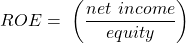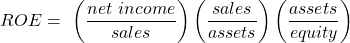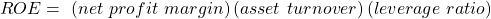Extended DuPont equation is: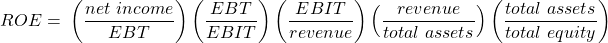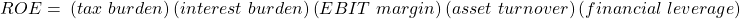An analyst has gathered the following information about a company:

• Operating profit margin = 12%
• Average tax rate = 30%
• Asset turnover ratio = 2 times
• Financial leverage multiplier = 1.5 times
• Interest burden = 0.6 times

Calculate the company’s ROE.

Solution: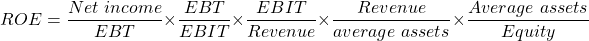Tax burden = 1 – tax rate = 1 – 0.3 = 0.7

ROE = 0.7 x 0.6 x 0.12 x 2 x 1.5 = 0.1512 = 15%

LIVE WEEKEND CLASSES *For 2024 Exam candidates*
This is default text for notification bar